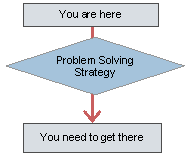HOME COURSES PREVIEW REVIEW ABOUT CONTACTTOLL-FREE INFO & ORDERING: M-F: 9am-5pm (PST): (877) RAPID-10

 Quick Search: Keywords:

 Rapid Learning Member Area:Note: If you are a legacy user of chemistry24 members, please request a new login access to the premium server with your full name and old login email via vip@rapidlearningcenter.com

 Rapid Courses Catalog : Physics in 24 Hours Chemistry in 24 Hours Biology in 24 Hours Mathematics in 24 Hours

Weekly Physics Tips:
Want to become a top gun in your class? How about study less yet score high? Sign up this Physics Survival Weekly to learn how. Designed specifically for students who are taking physics, this free newsletter will show you how to survive and excel in class! Weekly topics include:
• How to Study Physics Effectively
• How to Read Physics Textbooks Easily
• How to Solve Physics Problems Systematically
• How to Score High on Physics Exams Strategically
• How to Master Physics Rapidly
Each week, you will receive study tips on the topics above and visual tutorial or study template to enhance your physics learning. Enter your name and email below to subscribe free:

 Physics Study Lounge These study sheets are for quick review on the subjects. Refer to our rapid courses for comprehensive review.     - Basic Skills in Physics     - Basic Math for Physics     - How to Solve Physics Problems     - Newton's Laws Study Guide     - ElectroMagnetism Quick Review     - Atomic Physics At-A-Glance     - Core Concepts in Nuclear Physics     - Special Relativity Overview

 Other Related Sites
 Note: For course links to launch, disable popup blockers or hold the ctrl key while clicking the link.

How to Solve Physics Problems

What is problem-solving?General Problem-Solving Strategy

Step 1: Identify what’s being given

Step 2: Clarify what’s being asked.

If necessary, rephrase the question

Step 3: Select a strategy

Trial & error, search, deductive reasoning, knowledge-based, working backwards

Step 4: Solve using the strategy

Example:

Determine the relationship between force of friction and the coefficient of friction

 Step Description Information 1 Identify what’s given Force of friction and coefficient of friction 2 Identify what’s asked How they relate 3 Strategy: Deductive reasoning Force of friction is found with the equation: Ff = mFN m is the coefficient of friction and is a measure of the roughness of the two surfaces. 4 Apply the strategy As roughness increases, there is more friction. If m is the measure of the roughness of the surfaces, then as the roughness increases the m increases.  m and friction are directly related. 5 Review the solution The mathematic formula matches the reasoning.

Using symbols with mneumonics:

• When writing symbols in physics, use a mneumonic to help you remember what the symbol is representing.
•
• e.g.  For mass of proton, use “mp” and for velocity of car and truck use “Vc” and “Vt” rather than “V1” and “V2”
KUDOS Method

Use the KUDOS method for solving word problems.

• K = Known
• U = Unknown
• D = Definition
• O = Output
• S = Substantiation

Tips for KUDOS steps

• K (Known)
Use units to indetify information
Write information symbolically
Look for implied information
Write out chemical equations
• U (Unknown)
What is the problem looking for?
Write information symbolically
• D (Definition)
Find equalities to conver
Choose & Re-arrange equations
Look for missing information in other places
If you cannot find enough information, re-evaluate your plan
• O (Output)
Plug in values to the equations (use constants as needed)
Check unit cancellation & perform the calculation
• S (Substantiation)
Check units
Check signficiant figures
• Example:
• What is the change in velocity if the initial velocity is 23 m/s and the final velocity is 15 m/s?
 Step Source Information Write down K Initial velocity V0 = 23 m/s Final velocity V1 = 15 m/s U What is the change in velocity DV = ? m/s D Change in equation DV = V1 – V0 O Output of the equation DV = V1 – V0 DV = 15 m/s – 23 m/s DV = -8 m/s S Substantiation -8 m/s atm is reasonable for velocity “m/s” is the pressure unit given in the problem. 0 decimal places given 0 in answer

Exam prep tips

• Stay ahead of the game
• Make a cheat-sheet
• Know the format of the test and information that’s fair game
• Make a mock exam
• Attend the review session
• Get help early

Exam taking tips

• Arrive early and prepared
• Listen & Read instructions carefully
• Memory dump first
• Skim the test and form a plan